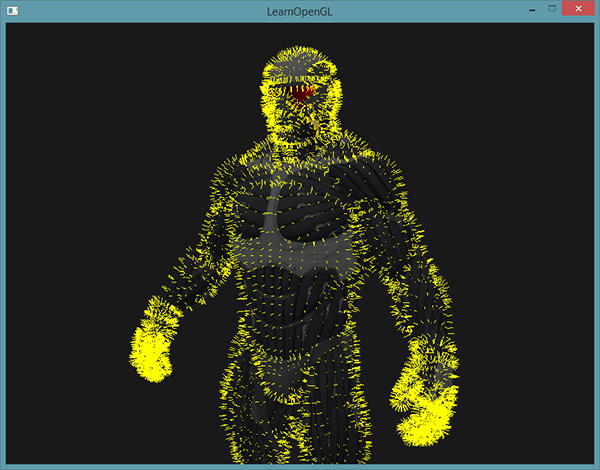# 几何着色器

#version 330 core
layout (points) in;
layout (line_strip, max_vertices = 2) out;

void main() {
gl_Position = gl_in.gl_Position + vec4(-0.1, 0.0, 0.0, 0.0);
EmitVertex();

gl_Position = gl_in.gl_Position + vec4( 0.1, 0.0, 0.0, 0.0);
EmitVertex();

EndPrimitive();
}


• points：绘制GL_POINTS图元时（1）。
• lines：绘制GL_LINESGL_LINE_STRIP时（2）
• lines_adjacencyGL_LINES_ADJACENCYGL_LINE_STRIP_ADJACENCY（4）
• trianglesGL_TRIANGLESGL_TRIANGLE_STRIPGL_TRIANGLE_FAN（3）
• triangles_adjacencyGL_TRIANGLES_ADJACENCYGL_TRIANGLE_STRIP_ADJACENCY（6）

• points
• line_strip
• triangle_stripin gl_Vertex
{
vec4  gl_Position;
float gl_PointSize;
float gl_ClipDistance[];
} gl_in[];


void main() {
gl_Position = gl_in.gl_Position + vec4(-0.1, 0.0, 0.0, 0.0);
EmitVertex();

gl_Position = gl_in.gl_Position + vec4( 0.1, 0.0, 0.0, 0.0);
EmitVertex();

EndPrimitive();
}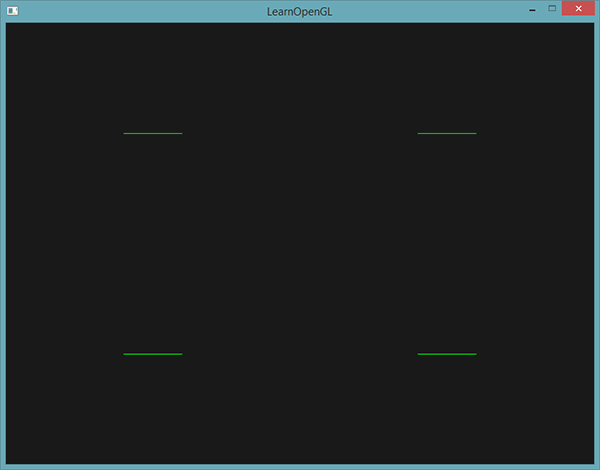glDrawArrays(GL_POINTS, 0, 4);


## 使用几何着色器

float points[] = {
-0.5f,  0.5f, // 左上
0.5f,  0.5f, // 右上
0.5f, -0.5f, // 右下
-0.5f, -0.5f  // 左下
};


#version 330 core
layout (location = 0) in vec2 aPos;

void main()
{
gl_Position = vec4(aPos.x, aPos.y, 0.0, 1.0);
}


#version 330 core
out vec4 FragColor;

void main()
{
FragColor = vec4(0.0, 1.0, 0.0, 1.0);
}


shader.use();
glBindVertexArray(VAO);
glDrawArrays(GL_POINTS, 0, 4);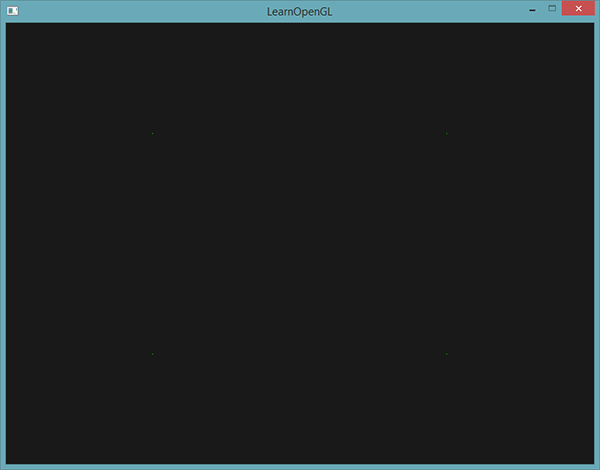#version 330 core
layout (points) in;
layout (points, max_vertices = 1) out;

void main() {
gl_Position = gl_in.gl_Position;
EmitVertex();
EndPrimitive();
}


geometryShader = glCreateShader(GL_GEOMETRY_SHADER);
...## 造几个房子

OpenGL中，三角形带(Triangle Strip)是绘制三角形更高效的方式，它使用顶点更少。在第一个三角形绘制完之后，每个后续顶点将会在上一个三角形边上生成另一个三角形：每3个临近的顶点将会形成一个三角形。如果我们一共有6个构成三角形带的顶点，那么我们会得到这些三角形：(1, 2, 3)、(2, 3, 4)、(3, 4, 5)和(4, 5, 6)，共形成4个三角形。一个三角形带至少需要3个顶点，并会生成N-2个三角形。使用6个顶点，我们创建了6-2 = 4个三角形。下面这幅图展示了这点：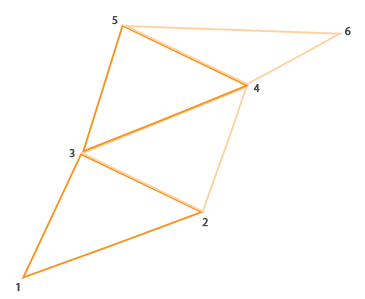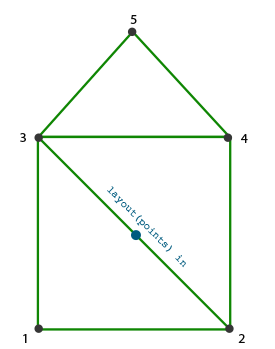#version 330 core
layout (points) in;
layout (triangle_strip, max_vertices = 5) out;

void build_house(vec4 position)
{
gl_Position = position + vec4(-0.2, -0.2, 0.0, 0.0);    // 1:左下
EmitVertex();
gl_Position = position + vec4( 0.2, -0.2, 0.0, 0.0);    // 2:右下
EmitVertex();
gl_Position = position + vec4(-0.2,  0.2, 0.0, 0.0);    // 3:左上
EmitVertex();
gl_Position = position + vec4( 0.2,  0.2, 0.0, 0.0);    // 4:右上
EmitVertex();
gl_Position = position + vec4( 0.0,  0.4, 0.0, 0.0);    // 5:顶部
EmitVertex();
EndPrimitive();
}

void main() {
build_house(gl_in.gl_Position);
}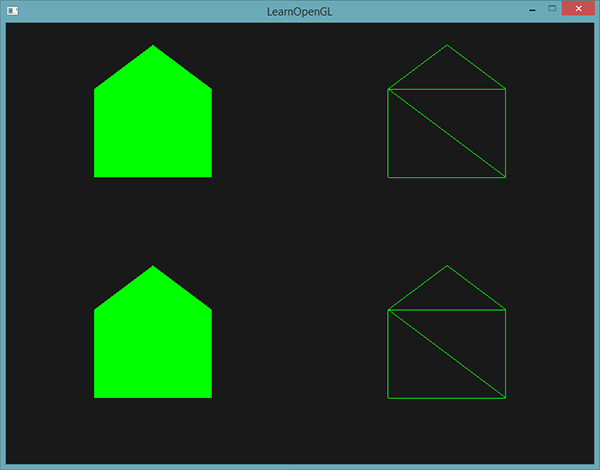float points[] = {
-0.5f,  0.5f, 1.0f, 0.0f, 0.0f, // 左上
0.5f,  0.5f, 0.0f, 1.0f, 0.0f, // 右上
0.5f, -0.5f, 0.0f, 0.0f, 1.0f, // 右下
-0.5f, -0.5f, 1.0f, 1.0f, 0.0f  // 左下
};


#version 330 core
layout (location = 0) in vec2 aPos;
layout (location = 1) in vec3 aColor;

out VS_OUT {
vec3 color;
} vs_out;

void main()
{
gl_Position = vec4(aPos.x, aPos.y, 0.0, 1.0);
vs_out.color = aColor;
}


in VS_OUT {
vec3 color;
} gs_in[];


Important

in vec3 vColor[];


out vec3 fColor;


fColor = gs_in.color; // gs_in 因为只有一个输入顶点
gl_Position = position + vec4(-0.2, -0.2, 0.0, 0.0);    // 1:左下
EmitVertex();
gl_Position = position + vec4( 0.2, -0.2, 0.0, 0.0);    // 2:右下
EmitVertex();
gl_Position = position + vec4(-0.2,  0.2, 0.0, 0.0);    // 3:左上
EmitVertex();
gl_Position = position + vec4( 0.2,  0.2, 0.0, 0.0);    // 4:右上
EmitVertex();
gl_Position = position + vec4( 0.0,  0.4, 0.0, 0.0);    // 5:顶部
EmitVertex();
EndPrimitive();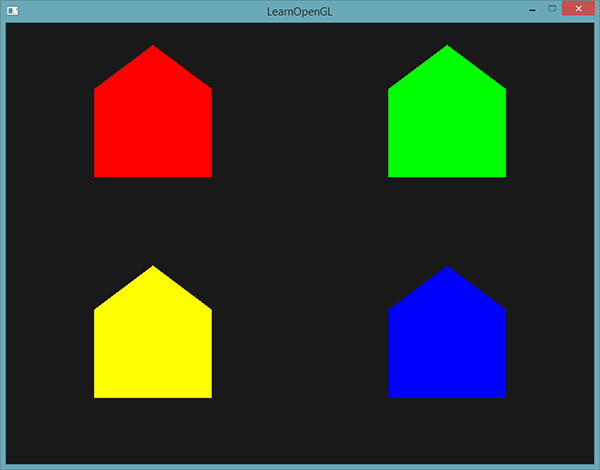fColor = gs_in.color;
gl_Position = position + vec4(-0.2, -0.2, 0.0, 0.0);    // 1:左下
EmitVertex();
gl_Position = position + vec4( 0.2, -0.2, 0.0, 0.0);    // 2:右下
EmitVertex();
gl_Position = position + vec4(-0.2,  0.2, 0.0, 0.0);    // 3:左上
EmitVertex();
gl_Position = position + vec4( 0.2,  0.2, 0.0, 0.0);    // 4:右上
EmitVertex();
gl_Position = position + vec4( 0.0,  0.4, 0.0, 0.0);    // 5:顶部
fColor = vec3(1.0, 1.0, 1.0);
EmitVertex();
EndPrimitive();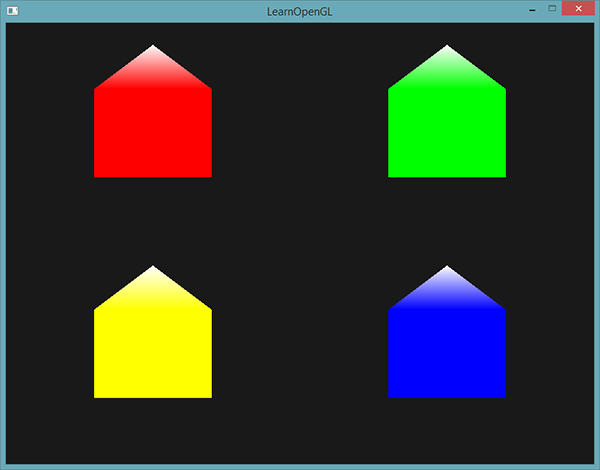# 爆破物体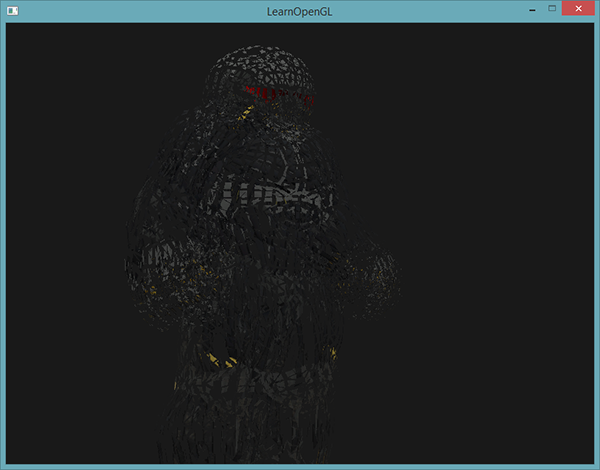vec3 GetNormal()
{
vec3 a = vec3(gl_in.gl_Position) - vec3(gl_in.gl_Position);
vec3 b = vec3(gl_in.gl_Position) - vec3(gl_in.gl_Position);
return normalize(cross(a, b));
}


vec4 explode(vec4 position, vec3 normal)
{
float magnitude = 2.0;
vec3 direction = normal * ((sin(time) + 1.0) / 2.0) * magnitude;
return position + vec4(direction, 0.0);
}


#version 330 core
layout (triangles) in;
layout (triangle_strip, max_vertices = 3) out;

in VS_OUT {
vec2 texCoords;
} gs_in[];

out vec2 TexCoords;

uniform float time;

vec4 explode(vec4 position, vec3 normal) { ... }

vec3 GetNormal() { ... }

void main() {
vec3 normal = GetNormal();

gl_Position = explode(gl_in.gl_Position, normal);
TexCoords = gs_in.texCoords;
EmitVertex();
gl_Position = explode(gl_in.gl_Position, normal);
TexCoords = gs_in.texCoords;
EmitVertex();
gl_Position = explode(gl_in.gl_Position, normal);
TexCoords = gs_in.texCoords;
EmitVertex();
EndPrimitive();
}


shader.setFloat("time", glfwGetTime());


# 法向量可视化

shader.use();
DrawScene();
DrawScene();


#version 330 core
layout (location = 0) in vec3 aPos;
layout (location = 1) in vec3 aNormal;

out VS_OUT {
vec3 normal;
} vs_out;

uniform mat4 view;
uniform mat4 model;

void main()
{
gl_Position = view * model * vec4(aPos, 1.0);
mat3 normalMatrix = mat3(transpose(inverse(view * model)));
vs_out.normal = normalize(vec3(vec4(normalMatrix * aNormal, 0.0)));
}


#version 330 core
layout (triangles) in;
layout (line_strip, max_vertices = 6) out;

in VS_OUT {
vec3 normal;
} gs_in[];

const float MAGNITUDE = 0.4;

uniform mat4 projection;

void GenerateLine(int index)
{
gl_Position = projection * gl_in[index].gl_Position;
EmitVertex();
gl_Position = projection * (gl_in[index].gl_Position +
vec4(gs_in[index].normal, 0.0) * MAGNITUDE);
EmitVertex();
EndPrimitive();
}

void main()
{
GenerateLine(0); // 第一个顶点法线
GenerateLine(1); // 第二个顶点法线
GenerateLine(2); // 第三个顶点法线
}


#version 330 core
out vec4 FragColor;

void main()
{
FragColor = vec4(1.0, 1.0, 0.0, 1.0);
}